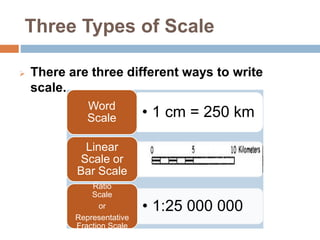# MAP SCALESA map represents a portion of the Earth’s surface. Since an accurate map represents the land, each map has a “scale” that indicates the relationship between a certain distance on the map and the distance on the ground. The map scale is usually located in the legend box of a map, which explains the symbols and provides other important information about the map. A map scale can be printed in a variety of ways;

• A ratio or representative fraction (RF) indicates how many units on the earth’s surface is equal to one unit on the map. It can be expressed as 1/100,000 or 1:100,000. In this example, one centimeter on the map equals 100,000 centimeters (1 kilometer) on the earth. It also means that one inch on the map is equal to 100,000 inches on the land (8,333 feet, 4 inches or about 1.6 miles). Or even 1 paperclip on the map is equal to 100,000 paperclips on the ground. Other common RFs include 1:63,360 (1 inch to 1 mile) and 1:1,000,000 (1 cm to 10 km).
• A word statement gives a written description of map distance, such as “One centimeter equals one kilometer” or “One centimeter equals ten kilometers.” Obviously, the first map would show much more detail than the second because one centimeter on the first map covers a much smaller area then on the second map.
• A graphic scale does solve this problem because it is simply a line marked with distance on the ground which the map user can use along with a ruler to determine scale on the map. In the U.S., a graphic scale often includes both metric and U.S. common units. As long as the size of the graphic scale is changed along with the map, it will be accurate.

Maps are often known as large scale or small scale. A large scale map refers to one which shows greater detail because the representative fraction (e.g. 1/25,000) is a larger fraction than a small scale map which would have an RF of 1/250,000 to 1/7,500,000. Large scale maps will have a RF of 1:50,000 or greater (i.e. 1:10,000). Those between 1:50,000 to 1:250,000 are maps with an intermediate scale. Maps of the world which fit on two 8 1/2 by 11 inch pages are very small scale, about 1 to 100 million.Maps aren’t just useful for directions, they can also help you determine the distance between two (or more) places .Here’s How to measure map distance:

1. Find the scale for the map you’re going to use – it might be a ruler-looking bar scale or a written scale, in words or numbers.
2. Use a ruler to measure the distance between the two places. If the line is quite curved, use a string to determine the distance and then measure the string.
1. If the scale is a representative fraction (and looks like 1/100,000 or 1:100,000), multiply the distance of the ruler by the denominator, giving distance in the ruler units.
2. If the scale is a word statement (i.e. “One centimeter equals one kilometer”) then determine the distance.
3. For a graphic scale, you’ll need to measure the graphic and divide the scale into the measured units on the ruler.

TEACHER´S CORNER:

lesson plans on map scales

POWER POINT:

Map scales

NOTES:

types of scale

Maps aren

WORKSHEETS TO PRACTICE:

map of the united states to practice map scales

using maps with diff scales

using-scale-map_WMZRF

VIDEOS TO REVIEW:

This site uses Akismet to reduce spam. Learn how your comment data is processed.